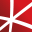# Top 20 NuGet numerics Packages

Foundational classes for financial, engineering, and scientific applications, including complex number classes, general vector and matrix classes, structured sparse matrix classes and factorizations, general sparse matrix classes and factorizations, general matrix decompositions, least squares solut...
The GPU-accelerated version of package CenterSpace.NMath. With a few minor exceptions, such as optional GPU configuration settings, the API is identical between CenterSpace.NMath.Premium and CenterSpace.NMath. If using at least .NET Framework 4.6.1 or .NET Core 2.0, we recommend using one of our NMa...
The Extreme Optimization Numerical Libraries for .NET are a set of libraries for numerical computing and data analysis. This is the main package that contains all the core functionality. For optimal performance, we strongly recommend also referencing one of the native packages based on Intel's Mat...
General purpose C# code library.
Foundational classes for financial, engineering, and scientific applications, including complex number classes, general vector and matrix classes, structured sparse matrix classes and factorizations, general sparse matrix classes and factorizations, general matrix decompositions, least squares solut...
Performance and memory optimised matrix algebra library based on cross platform MKL.NET. - Matrix expressions are optimised to perform intermediate calculations inplace and reuse memory. - Operations such as scale, transpose, +, * are combined into single MKL calls. - Intermediate matrices are disp...
Class library implementing advanced mathematical algorithms, transforms, and time series manipulations. Implementations favour simplicity and correctness.
Silk.NET is a high-speed, advanced library, providing bindings to popular low-level APIs such as OpenGL, OpenCL, OpenAL, OpenXR, GLFW, SDL, Vulkan, Assimp, and DirectX.
Foundational classes for financial, engineering, and scientific applications, including complex number classes, general vector and matrix classes, structured sparse matrix classes and factorizations, general sparse matrix classes and factorizations, general matrix decompositions, least squares solut...
Foundational classes for financial, engineering, and scientific applications, including complex number classes, general vector and matrix classes, structured sparse matrix classes and factorizations, general sparse matrix classes and factorizations, general matrix decompositions, least squares solut...## NMath - Standard Library - Windows and Linux - x64

Foundational classes for financial, engineering, and scientific applications, including complex number classes, general vector and matrix classes, structured sparse matrix classes and factorizations, general sparse matrix classes and factorizations, general matrix decompositions, least squares solut...## NMath - Standard Library - Windows - x86 and x64

Foundational classes for financial, engineering, and scientific applications, including complex number classes, general vector and matrix classes, structured sparse matrix classes and factorizations, general sparse matrix classes and factorizations, general matrix decompositions, least squares solut...
Statistical computations in F# using the Microsoft Solver Foundation
Foundational classes for financial, engineering, and scientific applications, including complex number classes, general vector and matrix classes, structured sparse matrix classes and factorizations, general sparse matrix classes and factorizations, general matrix decompositions, least squares solut...
A simple cross platform .NET API for Intel MKL. Reference the MKL.NET package and required runtime packages and use the static MKL functions. The correct native libraries will be included and loaded at runtime. Exposing functions from MKL keeping the syntax as close to the c developer reference as...## Steema Numerics Windows TeeChart

Steema Numerics is a new suite of .NET 5.0 assemblies designed to facilitate the integration of Machine Learning in the analaysis of your data.
Steema Numerics is a new suite of .NET 5.0 assemblies designed to facilitate the integration of Machine Learning in the analaysis of your data.
Silk.NET is a high-speed, advanced library, providing bindings to popular low-level APIs such as OpenGL, OpenCL, OpenAL, OpenXR, GLFW, SDL, Vulkan, Assimp, and DirectX.
Silk.NET is a high-speed, advanced library, providing bindings to popular low-level APIs such as OpenGL, OpenCL, OpenAL, OpenXR, GLFW, SDL, Vulkan, Assimp, and DirectX.
Silk.NET is a high-speed, advanced library, providing bindings to popular low-level APIs such as OpenGL, OpenCL, OpenAL, OpenXR, GLFW, SDL, Vulkan, Assimp, and DirectX.